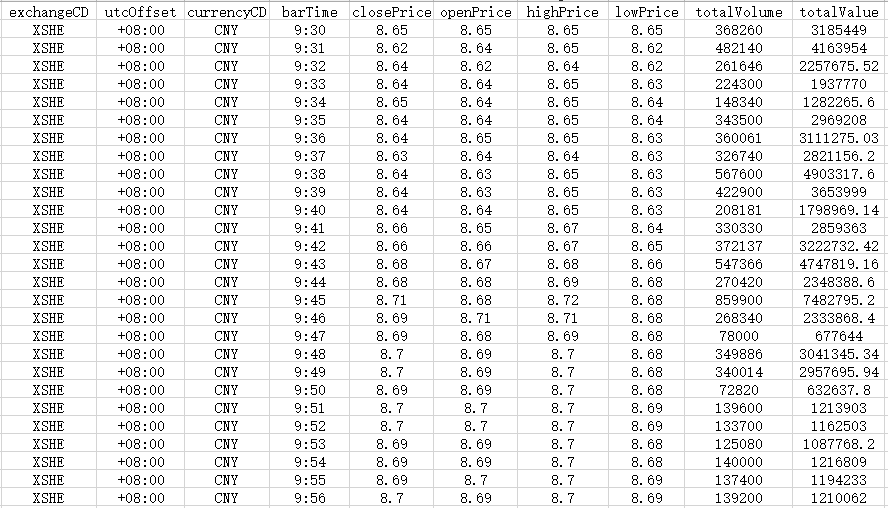# VWAP

VWAP是Volume Weighted Average Price的缩写，译为成交量加权平均价，VWAP策略是一种拆分大额委托单，在约定时间段内分批执行，以期使得最终买入或卖出成交均价尽量接近这段时间内整个市场成交均价的交易策略。它是量化交易系统中常用的一个基准。作为一个基准量，VWAP就是一个计算公式：

Vwap=ni=1priceivolumeini=1volumei

VWAP模型做到这一点的关键是历史成交量、未来成交量的预测、市场动态总成交量以及拆单的时间段（就是总共要将总单拆分成多少单分别以怎样的时间频率交易）。较为高级的VWAP模型要使用交易所单簿（Order Book）的详细信息，这要求系统能够得到即时的第二级市场数据（Level II Market Data）。
VWAP模型对于在几个小时内执行大单的效果最好。在交易量大的市场中，VWAP效果比在流动性差的市场中要好。在市场出现重要事件的时候往往效果不那么好。如果订单非常大，譬如超过市场日交易量的1%的话，即便VWAP可以在相当大的程度上改善市场冲击，但市场冲击仍然会以积累的方式改变市场，最终使得模型的效果差于预期。
VWAP算法交易的目的是最小化冲击成本，并不寻求最小化所有成本。理论上，在没有额外的信息，也没有针对股票价格趋势的预测的情况下，VWAP 是最优的算法交易策略。

# TWAP

TWAP交易时间加权平均价格Time Weighted Average Price 模型是把一个母单的数量平均地分配到一个交易时段上。该模型将交易时间进行均匀分割，并在每个分割节点上将拆分的订单进行提交。例如，可以将某个交易日的交易时间平均分为N 段，TWAP 策略会将该交易日需要执行的订单均匀分配在这N 个时间段上去执行，从而使得交易均价跟踪TWAP，也是一个计算公式：

Twap=ni=1pricein

TWAP不考虑交易量的因素。TWAP的基准是交易时段的平均价格，它试图付出比此时段内平均买卖差价小的代价执行一个大订单。TWAP模型设计的目的是使交易对市场影响减小的同时提供一个较低的平均成交价格，从而达到减小交易成本的目的。在分时成交量无法准确估计的情况下，该模型可以较好地实现算法交易的基本目的。但是使用TWAP过程中的一个问题是，在订单规模很大的情况下，均匀分配到每个节点上的下单量仍然较大，当市场流动性不足时仍可能对市场造成一定的冲击。另一方面，真实市场的成交量总是在波动变化的，将所有的订单均匀分配到每个节点上显然是不够合理的。因此，算法交易研究人员很快建立了基于成交量变动预测的VWAP 模型。不过，由于TWAP 操作和理解起来非常简单，因此其对于流动性较好的市场和订单规模较小的交易仍然适用。

# 代码实现C++

// calculate vwap value
double calc_vwap(std::vector<std::vector<std::string>> &marketDataTable)
{
int n = marketDataTable.size() - 1; // skip the first title line
double total_sum = 0.0;
int volume_sum = 0;
for (int i = 1; i <= n; i++)
{
// get the price and volume according to table structure
double high_price = atof(marketDataTable[i].c_str());
double low_price = atof(marketDataTable[i].c_str());
double price = (high_price + low_price) / 2;
int volume = atoi(marketDataTable[i].c_str());
// compute total sum and volume sum
total_sum += price * volume;
volume_sum += volume;
}

}

// calculate twap value
double calc_twap(std::vector<std::vector<std::string>> &marketDataTable)
{
int n = marketDataTable.size() - 1; // skip the first title line
double price_sum = 0.0;
for (int i = 1; i <= n; i++)
{
// get the price and volume according to table structure
double high_price = atof(marketDataTable[i].c_str());
double low_price = atof(marketDataTable[i].c_str());
double price = (high_price + low_price) / 2;
// compute price sum and time sum
// here use the 1 min K-line data, so total time is n minutes
price_sum += price;
}

return price_sum / n;
}

python

# calculate vwap value
def calc_vwap(marketDataTable):
n = len(marketDataTable) - 1
total_sum = 0.0
volume_sum = 0
for i in range(1, n + 1):
high_price = float(marketDataTable[i])
low_price = float(marketDataTable[i])
price = (high_price + low_price) / 2
volume = int(marketDataTable[i])
total_sum += price * volume
volume_sum += volume

# calculate vwap value
def calc_twap(marketDataTable):
n = len(marketDataTable) - 1
price_sum = 0.0
for i in range(1, n + 1):
high_price = float(marketDataTable[i])
low_price = float(marketDataTable[i])
price = (high_price + low_price) / 2
price_sum += price

return price_sum / n

reading market data
calculating TWAP and VWAP
VWAP: 8.66789
TWAP: 8.66475

csdn: twap/vwap code
github: twap/vwap code03-113万+
10-261万+
08-102万+
10-081万+
03-19
01-14610
01-14185
12-17202
06-28
03-19
11-04698
01-30421
02-06233
01-09236